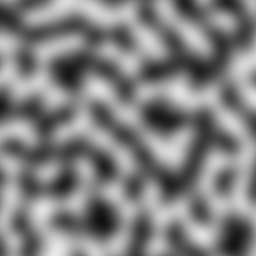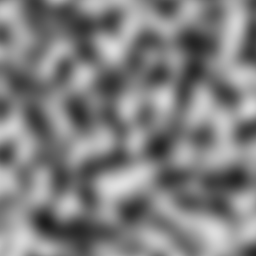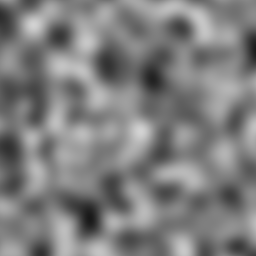# OpenSimplex Noise``````    OpenSimplex noise is an n-dimensional gradient noise function that was
developed in order to overcome the patent-related issues surrounding
Simplex noise, while continuing to also avoid the visually-significant
directional artifacts characteristic of Perlin noise.
``````

This is merely a python port of Kurt Spencer's original code (released to the public domain) and neatly wrapped up in a package.

# STATUS

Library is stable, it has pretty good test coverage but haven't gotten much optimizations.

Tested with Python 3.6, 3.7, 3.8 on Linux, MacOS and Windows

I like to think that the lack of recent commits is a sign of stable and feature complete code ;)

# USAGE

Initialization:

``````    >>> from opensimplex import OpenSimplex
>>> tmp = OpenSimplex()
>>> print (tmp.noise2d(x=10, y=10))
0.732051569572
``````

Optionally, the class accepts a seed value:

``````    >>> tmp = OpenSimplex(seed=1)
>>> print (tmp.noise2d(x=10, y=10))
-0.4790979022623557
``````

The seed must be a valid python number. It's used internally to generate some permutation arrays, which is used for the noise generation.

If it isn't provided the class will default to use 0 as the seed.

Available class methods:

OpenSimplex.noise2d(x, y)

Generate 2D OpenSimplex noise from X,Y coordinates.

OpenSimplex.noise3d(x, y, z)

Generate 3D OpenSimplex noise from X,Y,Z coordinates.

OpenSimplex.noise4d(x, y, z, w)

Generate 4D OpenSimplex noise from X,Y,Z,W coordinates.

Running tests and benchmarks:

First make a virtualenv and install the dev. requirements:

``````    virtualenv venv
source venv/bin/activate
pip install -r requirements.txt
``````

and then simply run the tests:

``````    make test
``````

or the basic benchmark:

``````    make benchmark
``````

# FAQ

• Is this relevantly different enough to avoid any real trouble with the original patent?

If you read the patent claims:

Claim #1 talks about the hardware-implementation-optimized gradient generator. Most software implementations of Simplex Noise don't use this anyway, and OpenSimplex Noise certainly doesn't.

Claim #2(&3&4) talk about using (x',y',z')=(x+s,y+s,z+s) where s=(x+y+z)/3 to transform the input (render space) coordinate onto a simplical grid, with the intention to make all of the "scissor-simplices" approximately regular. OpenSimplex Noise (in 3D) uses s=-(x+y+z)/6 to transform the input point to a point on the Simplectic honeycomb lattice so that the simplices bounding the (hyper)cubes at (0,0,..,0) and (1,1,...,1) work out to be regular. It then mathematically works out that s=(x+y+z)/3 is needed for the inverse transform, but that's performing a different (and opposite) function.

Claim #5(&6) are specific to the scissor-simplex lattice. Simplex Noise divides the (squashed) n-dimensional (hyper)cube into n! simplices based on ordered edge traversals, whereas OpenSimplex Noise divides the (stretched) n-dimensional (hyper)cube into n polytopes (simplices, rectified simplices, birectified simplices, etc.) based on the separation (hyper)planes at integer values of (x'+y'+z'+...).

Another interesting point is that, if you read all of the claims, none of them appear to apply to the 2D analogue of Simplex noise so long as it uses a gradient generator separate from the one described in claim #1. The skew function in Claim #2 only applies to 3D, and #5 explicitly refers to n>=3.

And none of the patent claims speak about using surflets / "spherically symmetric kernels" to generate the "images with texture that do not have visible grid artifacts," which is probably the biggest similarity between the two algorithms.

# CREDITS

• Kurt Spencer - Original work
• A Svensson - Python port and package author
• CreamyCookie - Cleanup and optimizations
• Owen Raccuglia - Test cases
• /u/redblobgames - Fixed conversion for Java's long type, see Reddit
• PetyaVasya - Found bug with using c_long on Windows systems, see Issue #7

# LICENSE

While the original work was released to the public domain by Kurt, this package is using the MIT license. Please see the file LICENSE for details.

# Expected Output

2D noise (with default seed):{width="100px" height="100px"}

3D noise:{width="100px" height="100px"}

4D noise:{width="100px" height="100px"}

Get A Weekly Email With Trending Projects For These Topics
No Spam. Unsubscribe easily at any time.
python (54,453
noise (32